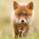920 visningar
EXPERIMENTAL:
similar to renko.
```//@version=2
study(title='[RS]Adaptive Range Candles V0', shorttitle='ARC', overlay=true)
multiplier = input(title='Range Multiplier:', type=float, defval=0.01)
SHOW_CLOSE_LINE = input(title='Show Close Line?', type=bool, defval=true)

h = na(h) ? high : high >= h ? high : h
l = na(l) ? low : low <= l ? low : l
range = (h-l)*multiplier

avg = na(avg) ? close : close > avg + range ? close : close < avg - range ? close : avg
pavg = change(avg) != 0 ? valuewhen(change(avg) != 0, avg, 1) : pavg

plotcandle(pavg, high, low, avg, color=avg > pavg ? lime : red)
plot(title='C', series=SHOW_CLOSE_LINE?close:na, color=black, linewidth=1, transp=0)```

## Kommentarersudhir.mehta
not possible in pinescript, as this is a overlay on top of already existing candles, for that it would need to be built in and replace the underlying candles.
Svara